2019-05-23 01:07:19 titing3539 阅读数 63

## 用梯度下降手写线性回归（只用numpy 、pandas）

• 用梯度下降方法预测PM2.5的值
李宏毅机器学习中的作业1 ，这里为了简化流程，突出方法，只用了train.csv数据。
简单说一下要干嘛：就是有很多条观测数据，每一条数据包含了24小时的观测数据，通过这些数据，预测未来PM2.5的值。

import pandas as pd
import numpy as np

train_data.insert(3, 'b', 1)    #  插入一列都为1的数， bias * 1  所有参数统一看成 w

a1 =  train_data[train_data['observation']=='PM2.5'].iloc[:-50,3:13] #用来训练参数 w
a2 =  train_data[train_data['observation']=='PM2.5'].iloc[-50:,3:13] # 后50行用来算下某参数下的error

a1_x = a1.values[:,:-1]   #前9个小时数据
a1_x_array = a_x.astype(np.float)
a1_y = a1.values[:,-1]    #第10个小时数据
a1_y_array = a_y.astype(np.float)

a2_x = a2.values[:,:-1]
a2_x_array=a2_x.astype(np.float)
a2_y = a2.values[:,-1]
a2_y_array=a2_y.astype(np.float)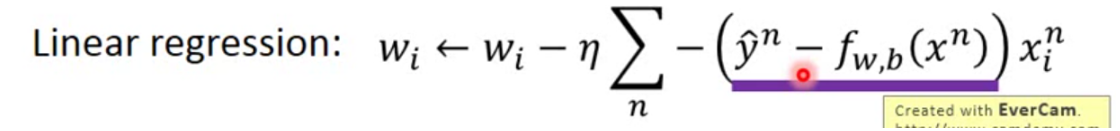#  线性回归 梯度下降
def update_w(dataMatin,label):
dataMatrix = np.mat(dataMatin)    # m 样本量 ，n 特征数量  (m , n)
labelMatrix = np.mat(label).transpose()   # (m , 1)
m, n = np.shape(dataMatrix)
alpha = 0.0000001        # 学习率
max_cycle = 100000000    #迭代次数
w = np.ones((n,1))          # w 初始值为1
for i in range(max_cycle):
h = dataMatrix*w           # （m ,n) × （n ,1) = (m , 1)    x*w
err = (labelMatrix - h)   #（m ,1)
w = w + alpha * dataMatrix.transpose()*err    # (n,m)×（m,1) = (n , 1)
return w

# 平方差的和 作为 error
def Error(y_label, y_fitting):
return np.sum(np.square(y_label,y_fitting))

# 测试集数据代入 训练好参数w的线性函数中的误差
def model_error(dataMatIn, label, w):
dataMatrix = np.mat(dataMatIn)
labelMatrix = np.mat(label).transpose()
h = (dataMatrix*w)
error = Error(labelMatrix, h)        #(m , 1)
return error


2019-05-21 14:02:10 weixin_44049128 阅读数 177

## 本博文主要是完成李宏毅机器学习HW1作业

### 作业规则

numpy> = 1.14
scipy == 1.2.1
pandas == 0.24.1。

• 选取合适的特征
• 实施线性回归
• 预测PM2.5的值

### 作业进行

#### 1 提取特征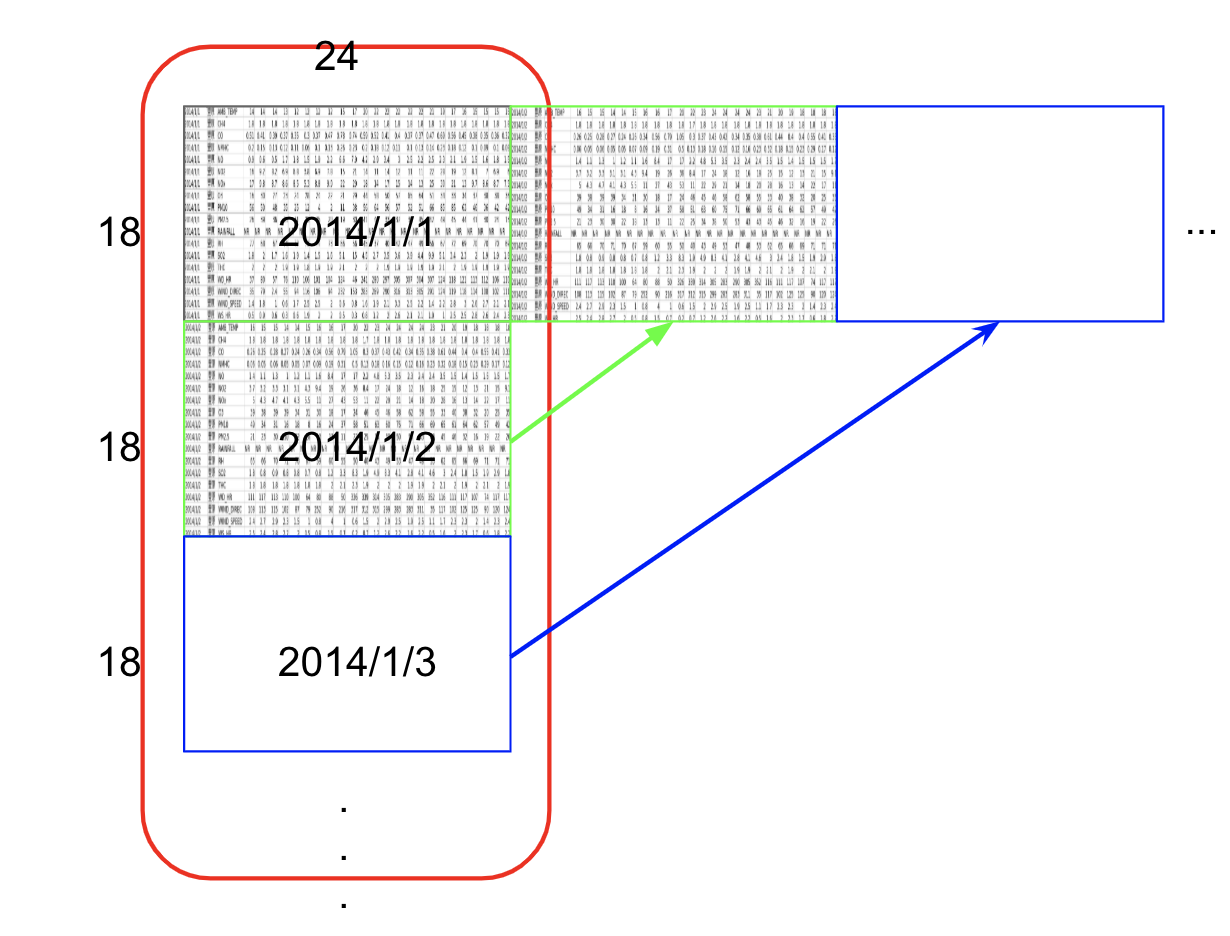train.csv:每个月前20天每个小时的气象资料(每小时有18种测试内容)。共12个月。

#### 2 伪代码

1. 处理数据，提取特征
train_x		# 前9小时数据
train_y		# 第10小时PM2.5数据

for i in all the given data:
sample every10 hrs：
train_x.append(previous 9-hr data)
train_y.append(the value of 10th-hr pm2.5)
add a bias term to every data in train_x

1. 实施线性回归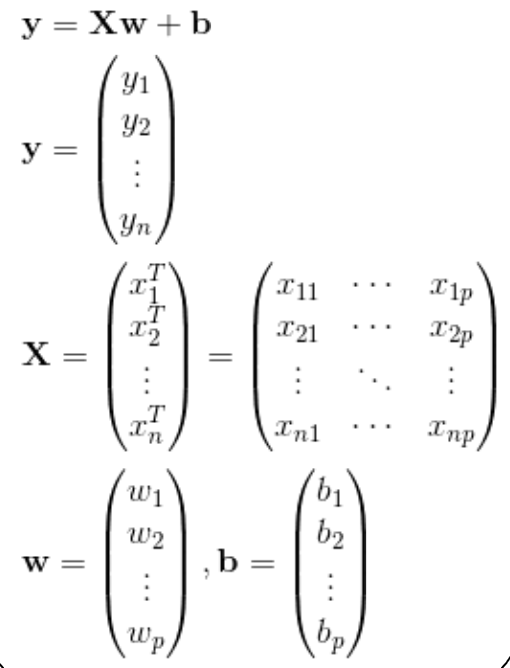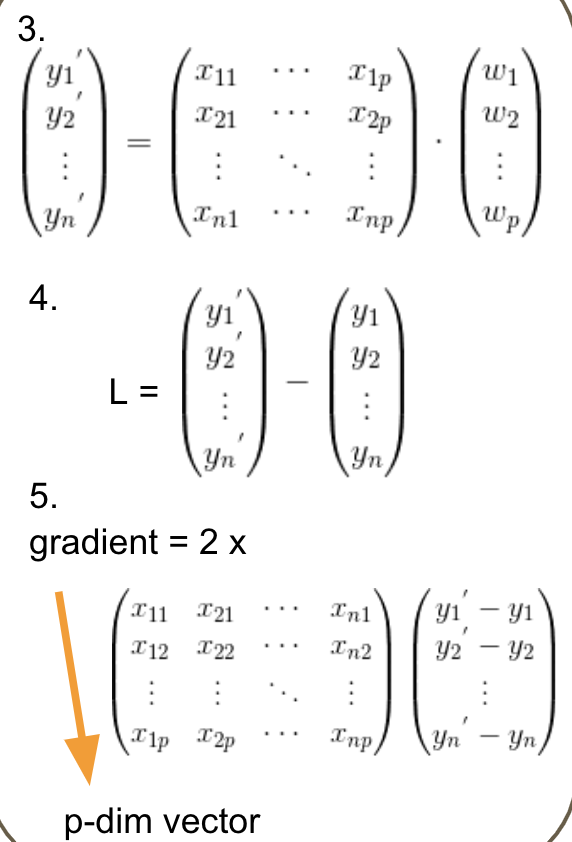Declare weight vector, initial lr ,and iteration	# 声明权重w 初始化学习率lr 以及训练轮数
for i_th iteration :
y’ = the product of train_x  and weight vector
Loss = y’ - train_y
gradient = 2 * np.dot((train_x)’, L )
prev_gra += gra**2

1. 预测PM2.5
read test_x.csv file	# 读取数据
for every 18 rows :
test_x.append()
test_x.append(9-hr data)
test_y = np.dot(weight vector, test_x)


#### 3 代码

#!/usr/bin/env python
# -*- coding: utf-8 -*-

import pandas as pd
import numpy as np

# 获取训练数据
def get_train_data():
'''
获取PM2.5数据：
train_data['observation']表示取csv数据中的“observation”列
返回：序号 污染物种类
train_data[train_data['observation'] == 'PM2.5']表示获取污染物中PM2.5的数据
iloc[:, 3:]表示取第3列之后的列以及所有行的数据
'''
data_pm25 = train_data[train_data['observation'] == 'PM2.5'].iloc[:, 3:]
x_list=[]
y_list=[]
for i in range(15):
# 获取前9小时pm2.5数据作为输入feature（并非只有0-8 1-9 2-10都可以）
x = data_pm25.iloc[:,i:i + 9]
x.columns = np.array(range(9))
# 获取第10小时pm2.5数据作为label
y = data_pm25.iloc[:, i + 9]
y.columns=
x_list.append(x)
y_list.append(y)

x_data = pd.concat(x_list)
y_data = pd.concat(y_list)

X = np.array(x_data, float)
# np.c_按行连接矩阵 给输入数据加bias
X = np.c_[np.ones((X.shape, 1)), X]
Y = np.array(y_data, float)
return X, Y

def get_test_data():
data_pm25 = test_data[test_data['AMB_TEMP'] == 'PM2.5'].iloc[:, 2:]
X = np.array(data_pm25, float)
X = np.c_[np.ones((X.shape, 1)), X]
return X

'''
w^{t+1} = w^t- eta/sqrt{sum^t_{i=0}(g^i)^2}g^t
'''
def __init__(self, lr=10, epochs=10000):
'''
:param lr: 学习率
:param epochs: 训练轮数
'''
self.lr = lr
self.epochs = epochs

def fit(self, x, y):
m = y.size
w = np.zeros(x.shape)
cost = []
for j in range(self.epochs):
# Model 模型
y_hat = np.dot(x, w)
# Error 误差
error = y_hat - y
grad = np.dot(x.transpose(), error) * 2
J = 1.0 / (m) * (np.sum(np.square(y_hat - y)))
cost.append(J)
return w, cost

X_train, y_train = get_train_data()
X_test = get_test_data()

w, cost = gd.fit(X_train, y_train)
print(w, cost[-1])

# 预测
h = np.dot(X_test,w)
erro = abs(h - real.value).sum() / len(real.value)
print('平均绝对值误差',erro)


2019-07-07 23:19:15 wangwei19871103 阅读数 98

# 神经网络可能也需要记忆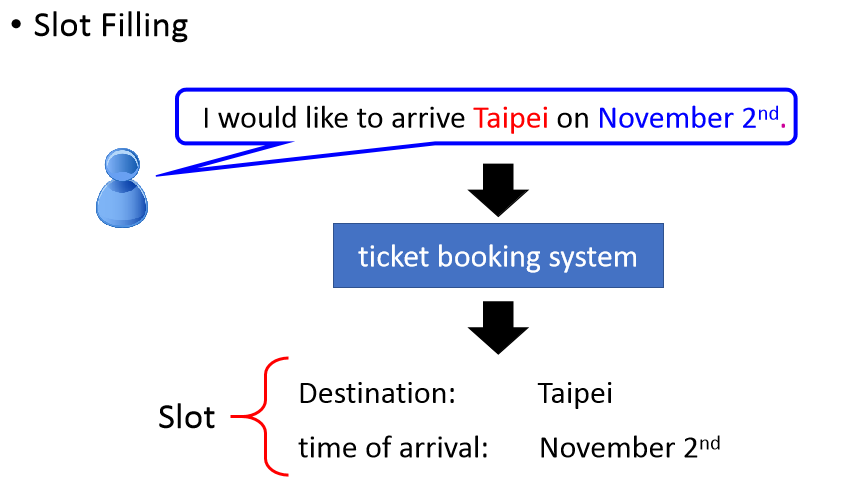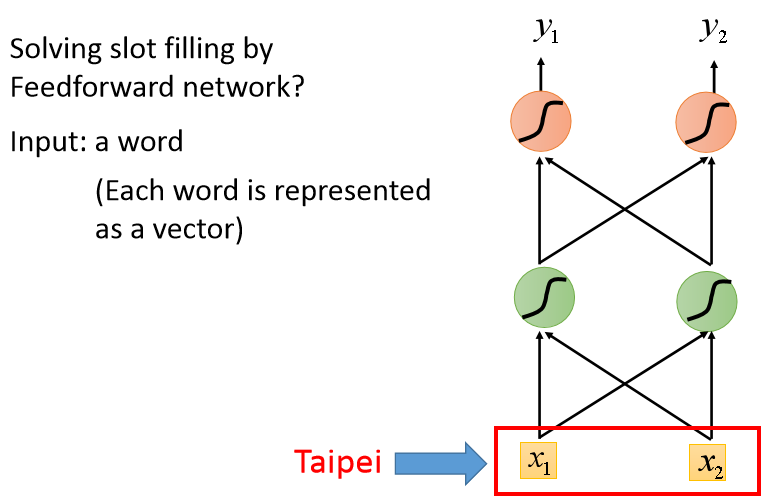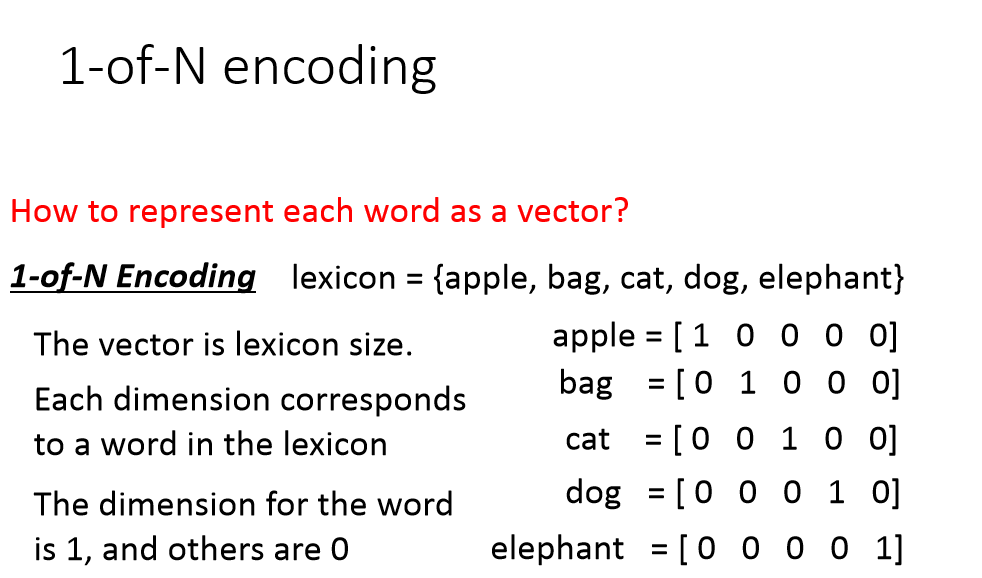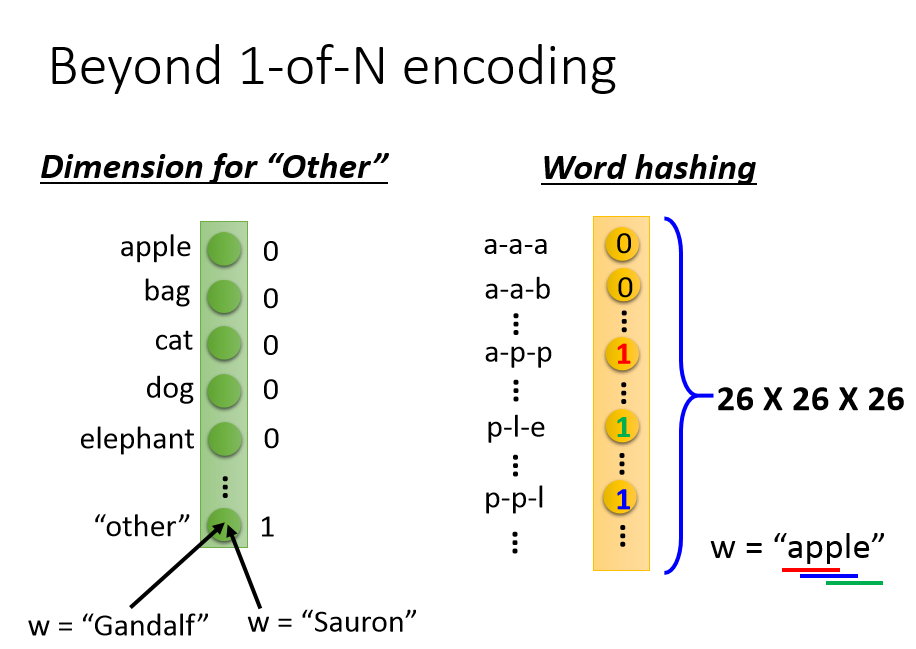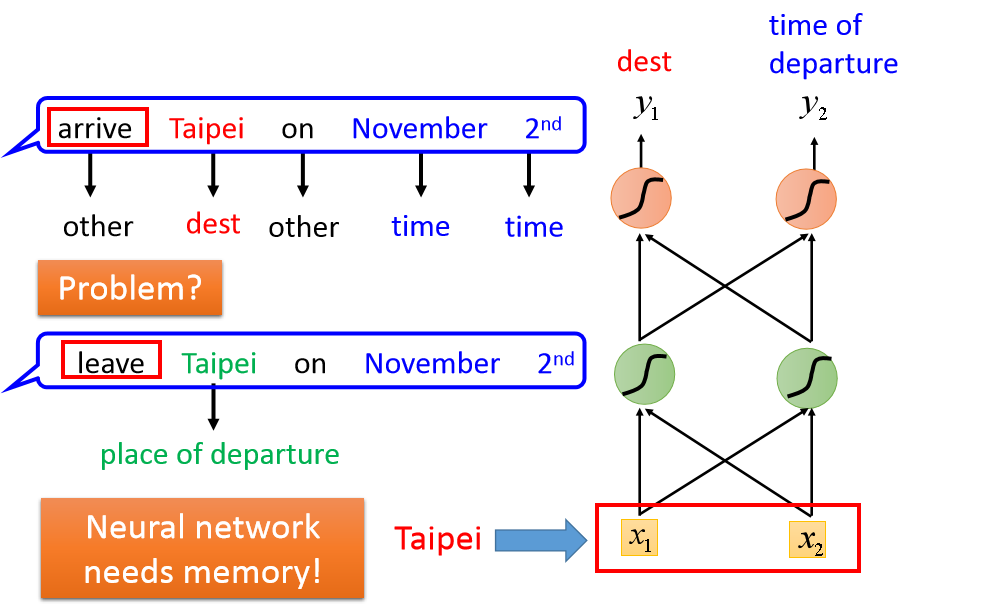# RNN简介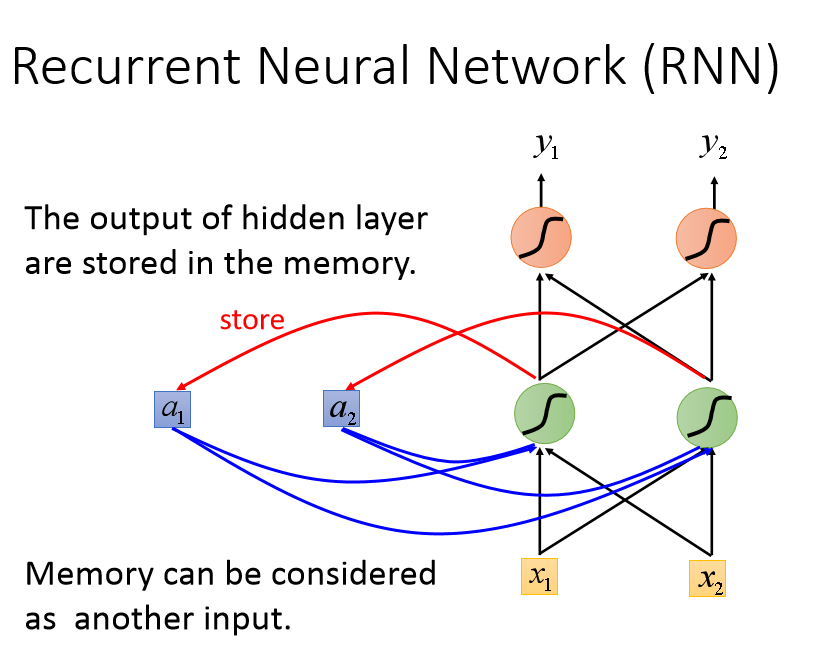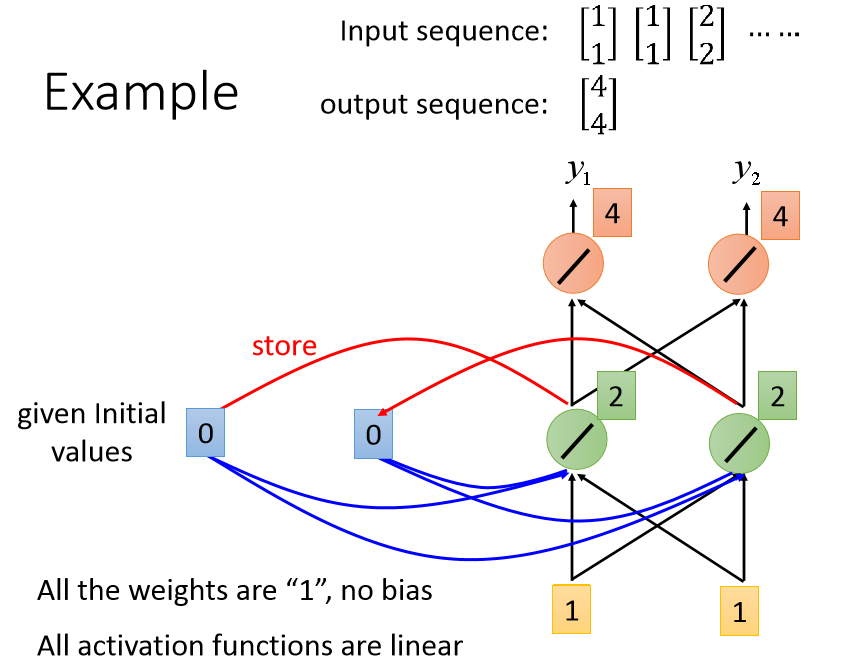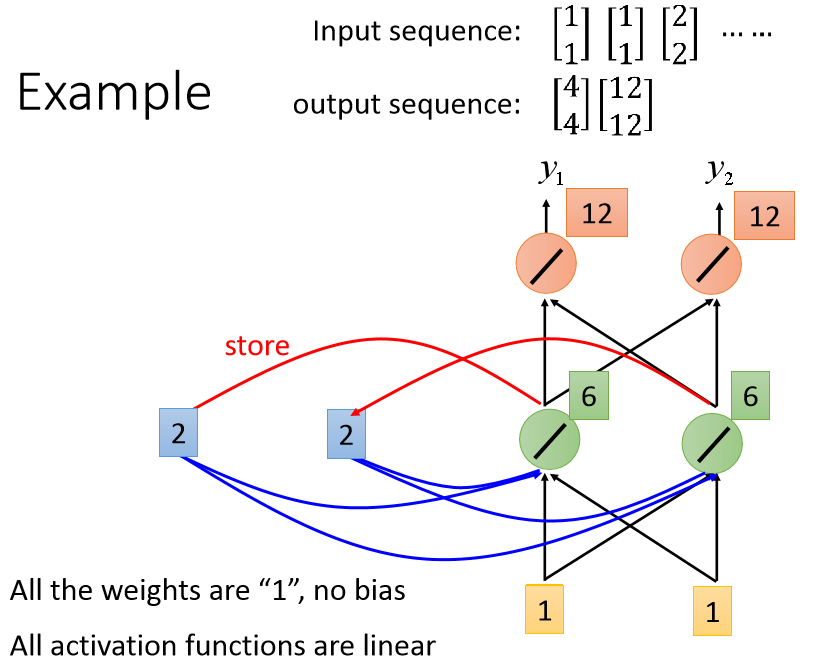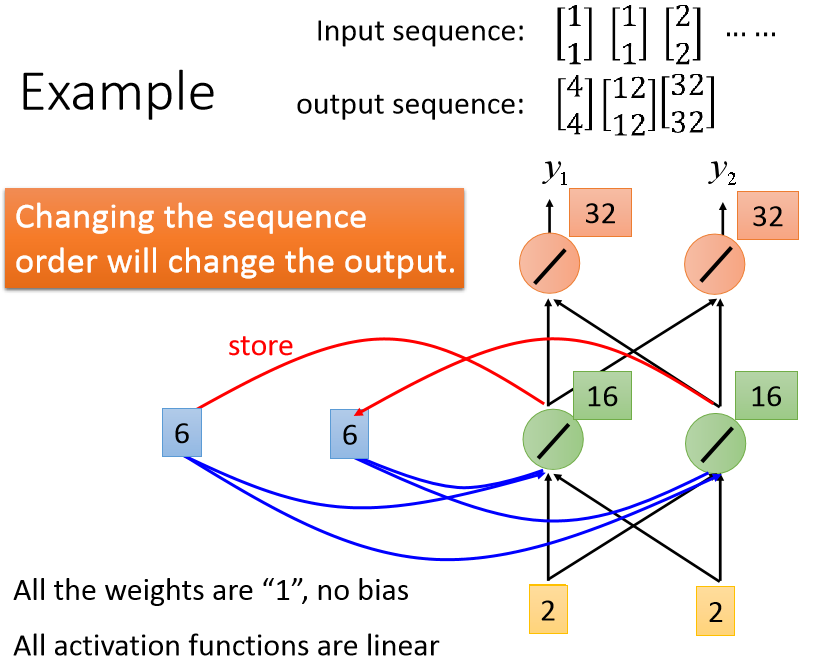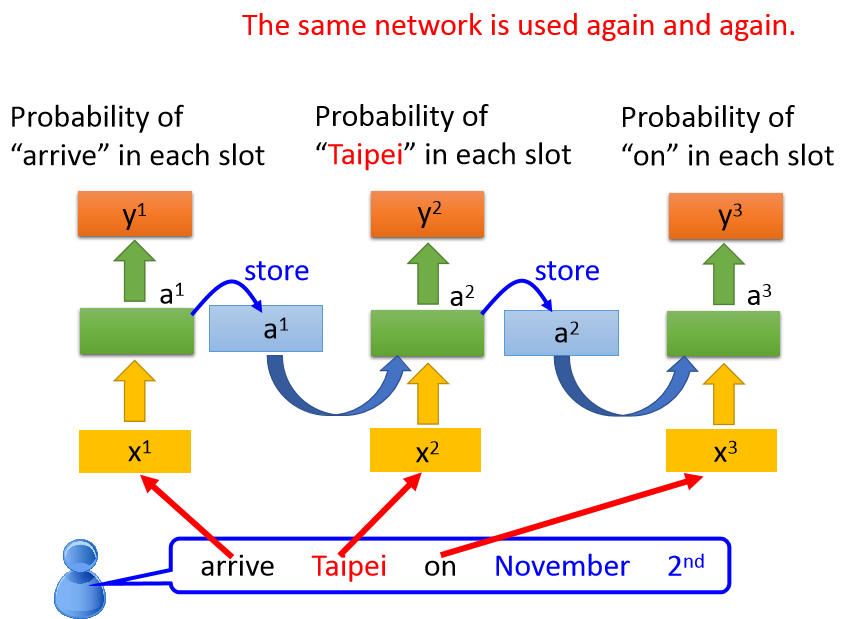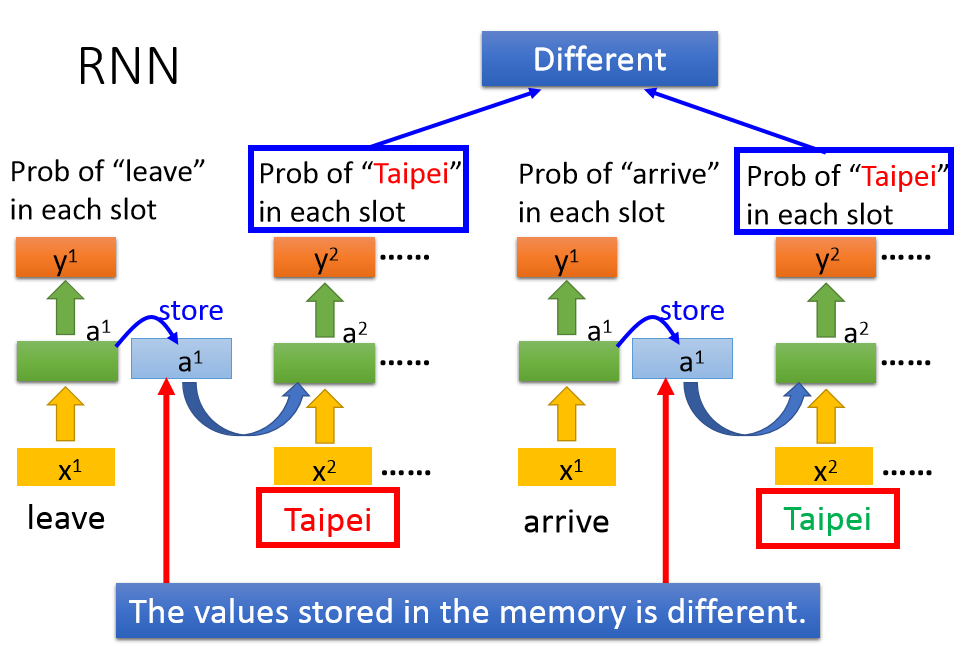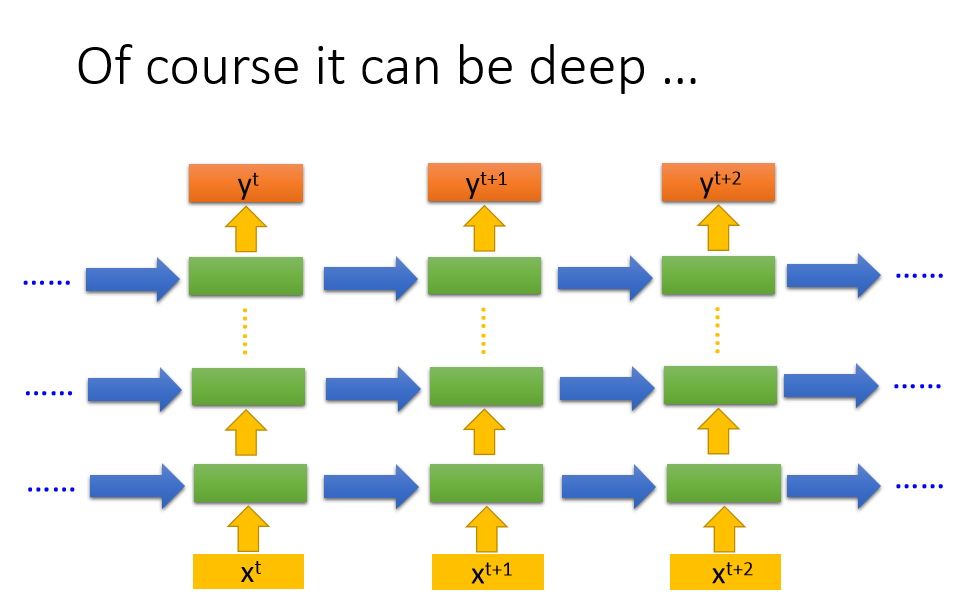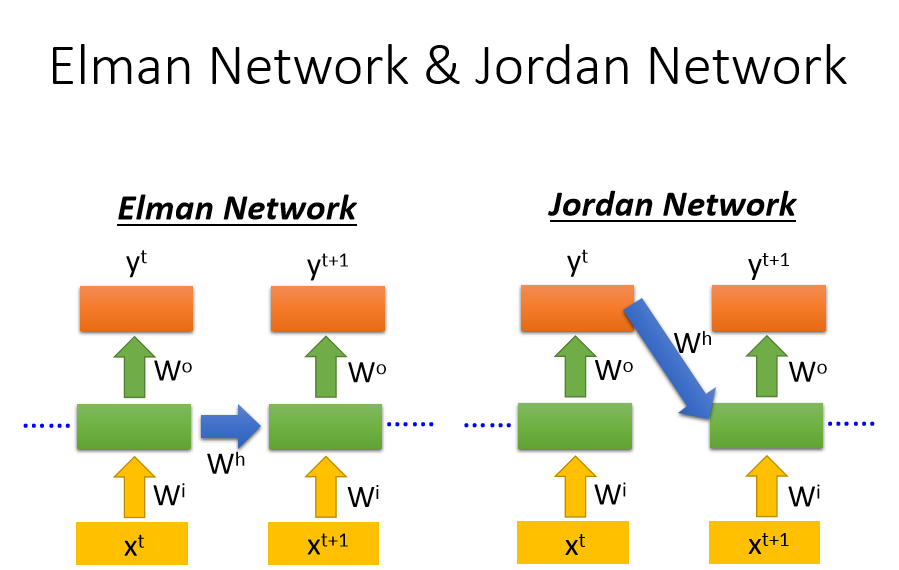# LSTM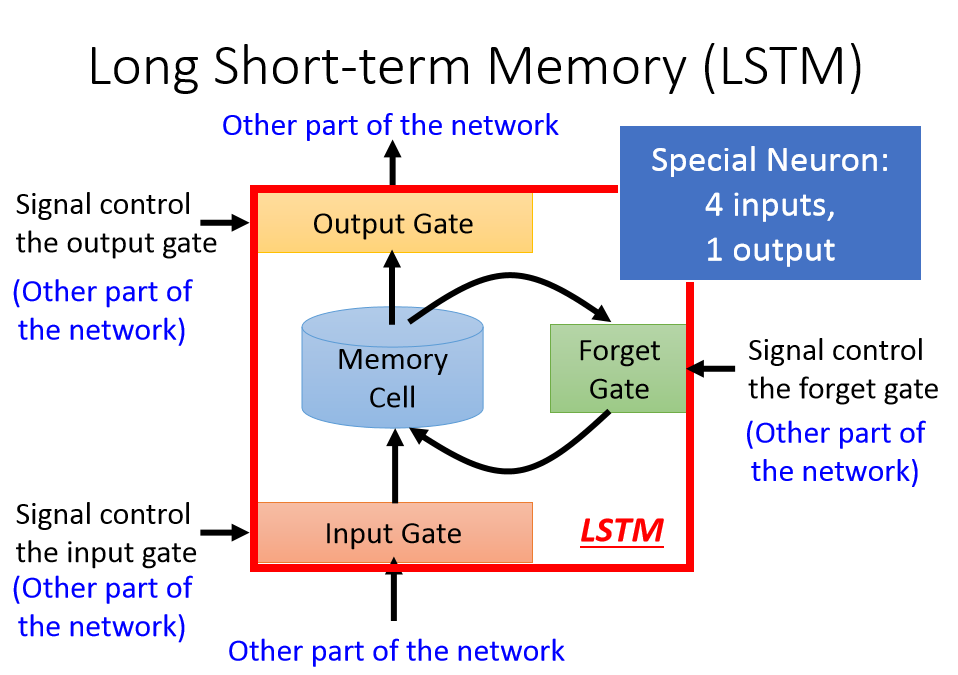LSTM就是长一点的短期记忆，前面介绍的简单的那种，基本就是保存了上一个时间的记忆，可能马上又被替换成新的了，LSTM是可以根据需要保存了时间稍微长一点的。当然最简单的RNN也可能出现梯度消失和梯度爆炸问题，最开始的记忆因为前向传播被乘以N个权重，反向传播链式法则最后传到前面的梯度可能是很小，也可能很大。LSTM的一个模块包括4个输入，1个输出，1个记忆单元。
4个输入为：
输入，此刻输入的单词
输入门，控制是否要使用输入
遗忘门，控制是否要把以前的记忆给抹去
输出门，控制是否将结果输出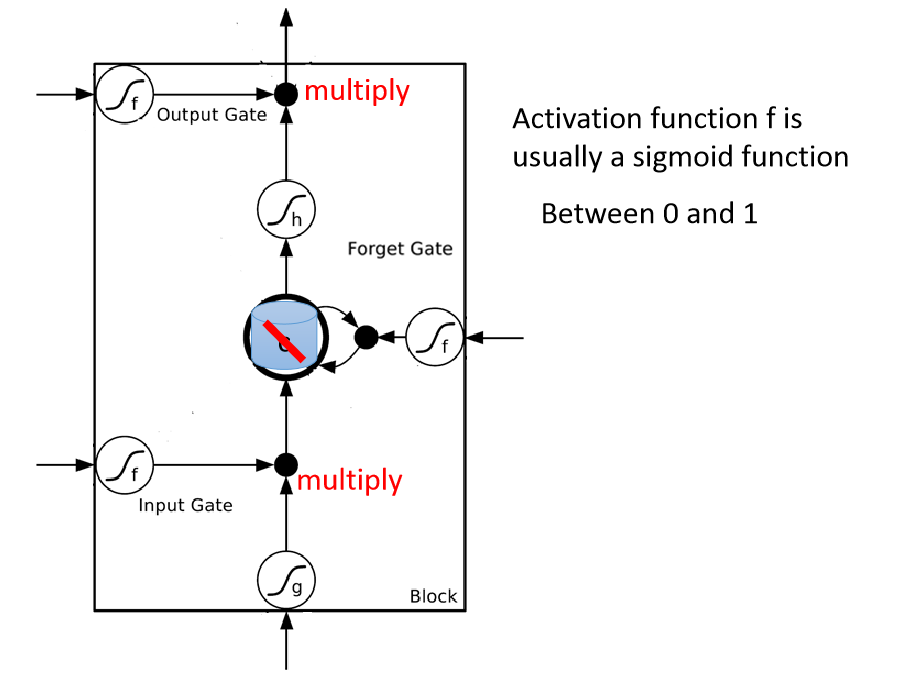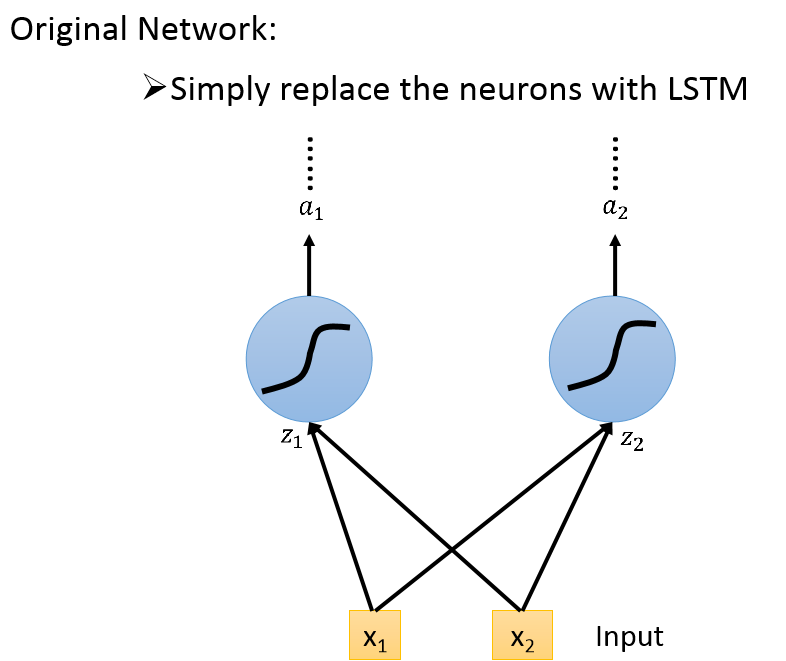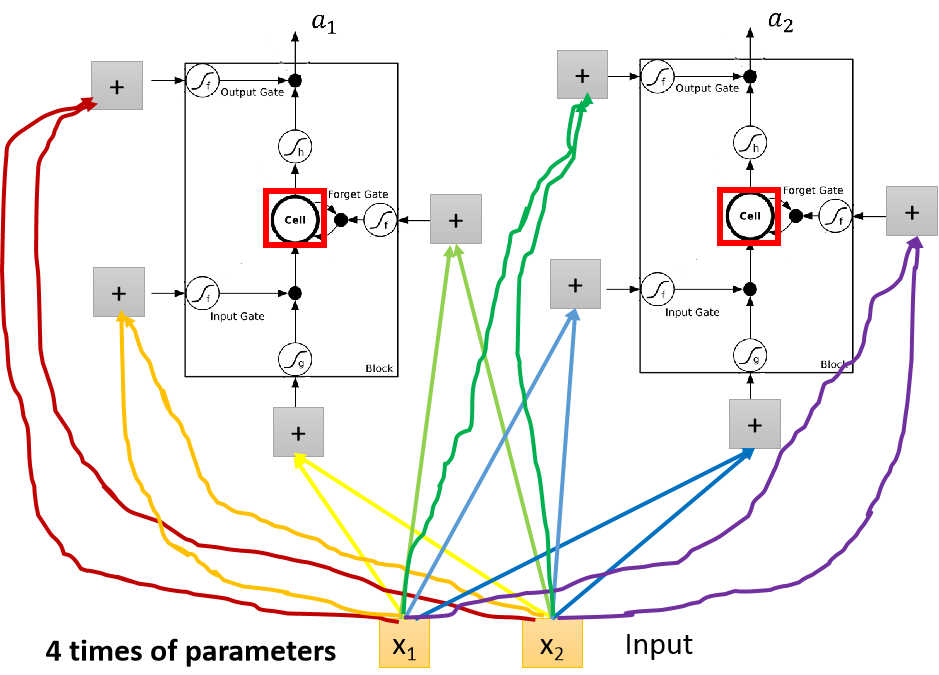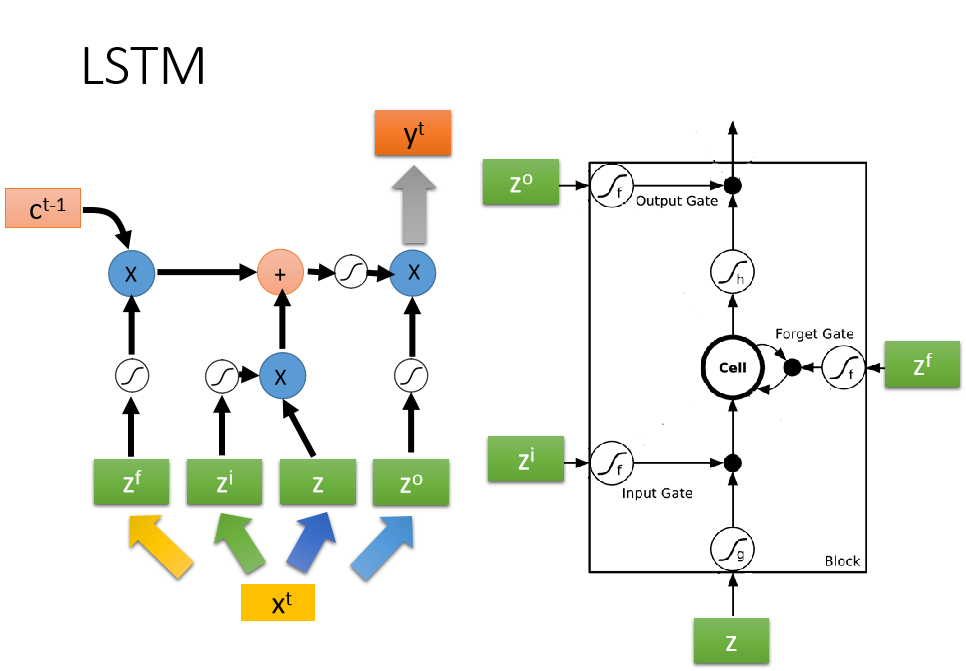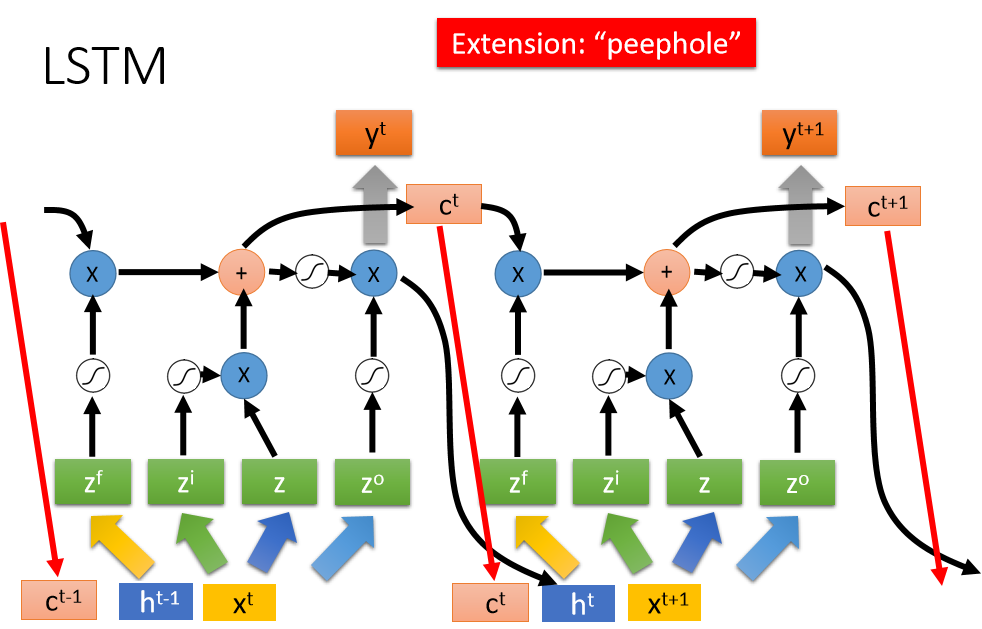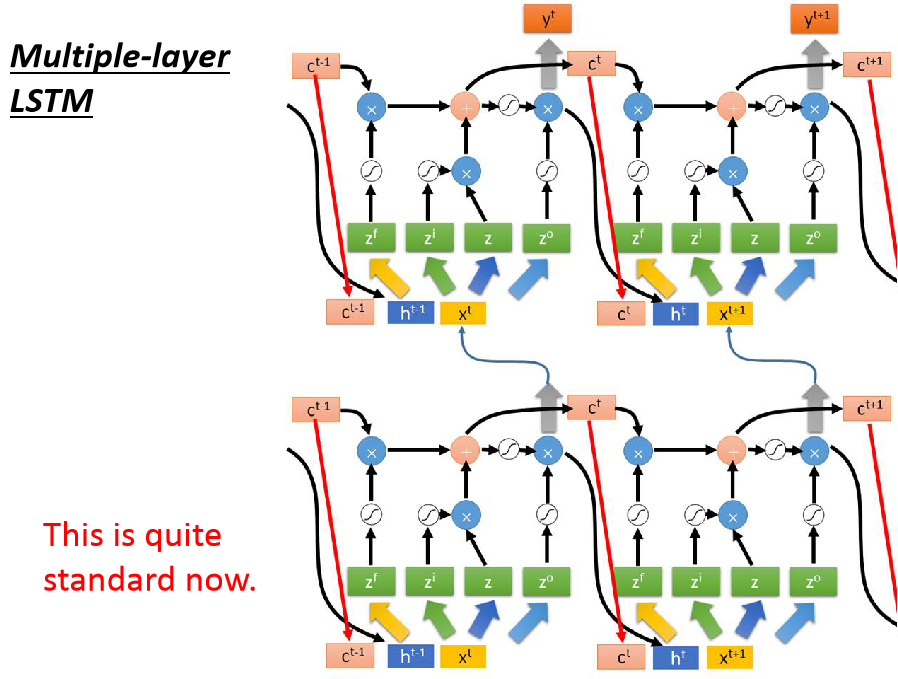# 总结

RNN就是让神经网络有记忆，以便需要有关联上下文的时候可以起作用，LSTM是一种RNN，可以保持记忆一定的时间。

2019-05-31 01:54:47 titing3539 阅读数 41

## 1、LR损失函数和梯度下降一般线性函数的损失函数是平方损失函数，LR求最大似然估计是因为sigmoid函数求导后无法保证是凸函数，在优化的过程中可能得到的是局部最小值，而非全局最优。取对数是方便求导。损失函数除以m这一因子并不改变最终求导极值结果，通过除以m可以得到平均损失值避免样本数量对于损失值的影响

## 2、Softmax原理

顾名思义，softmax由两个单词组成，其中一个是max。对于max我们都很熟悉，比如有两个变量a，b。如果a>b，则max为a，反之为b。
另一个单词是soft。max存在的一个问题是什么呢？如果将max看成一个分类问题，就是非黑即白，最后的输出是一个确定的变量。更多的时候，我们希望输出的是取到某个分类的概率，或者说我们希望分值大的那一项被经常取到，而分值较小的那一项也有一定的概率被取到，所以我们就应用到了soft的概念，即最后的输出是每个分类被取到的概率。下面的图就很容易理解Softmax原理：假设有一个数组V，Vi表示V中的第i个元素，那么这个元素的softmax值为:该元素的softmax值，就是该元素的指数与所有元素指数和的比值。

## 3、Softmax损失函数和梯度下降

softmax损失函数用的是交叉熵函数，主要是由于这个求导结果比较简单，易于计算，并且交叉熵解决某些损失函数学习缓慢的问题李宏毅机器学习 相关内容

2019-05-12 21:44:11 qq_38364952 阅读数 425

# 李宏毅机器学习第一次作业

## 3. 线性回归 Loss Function 推导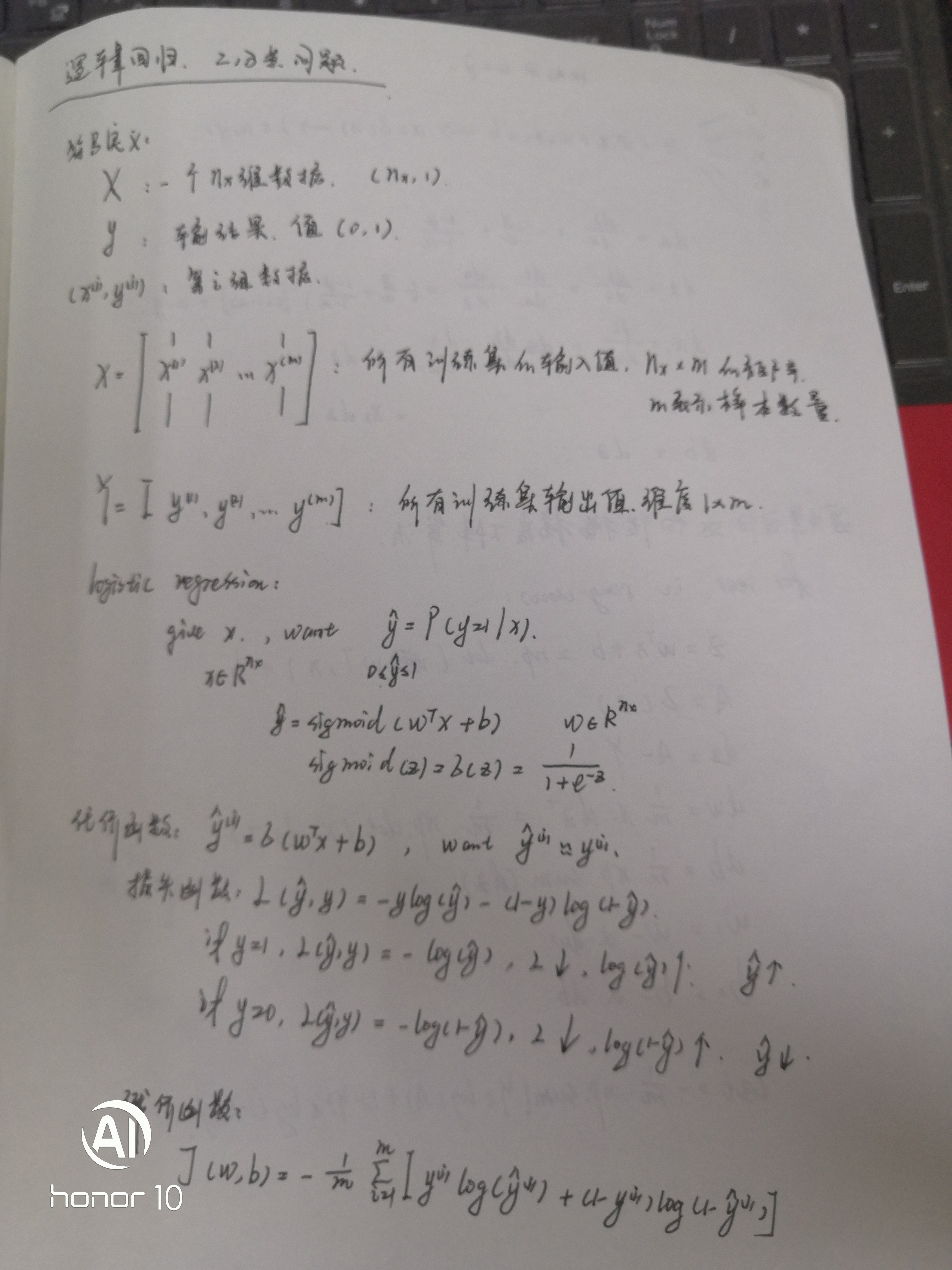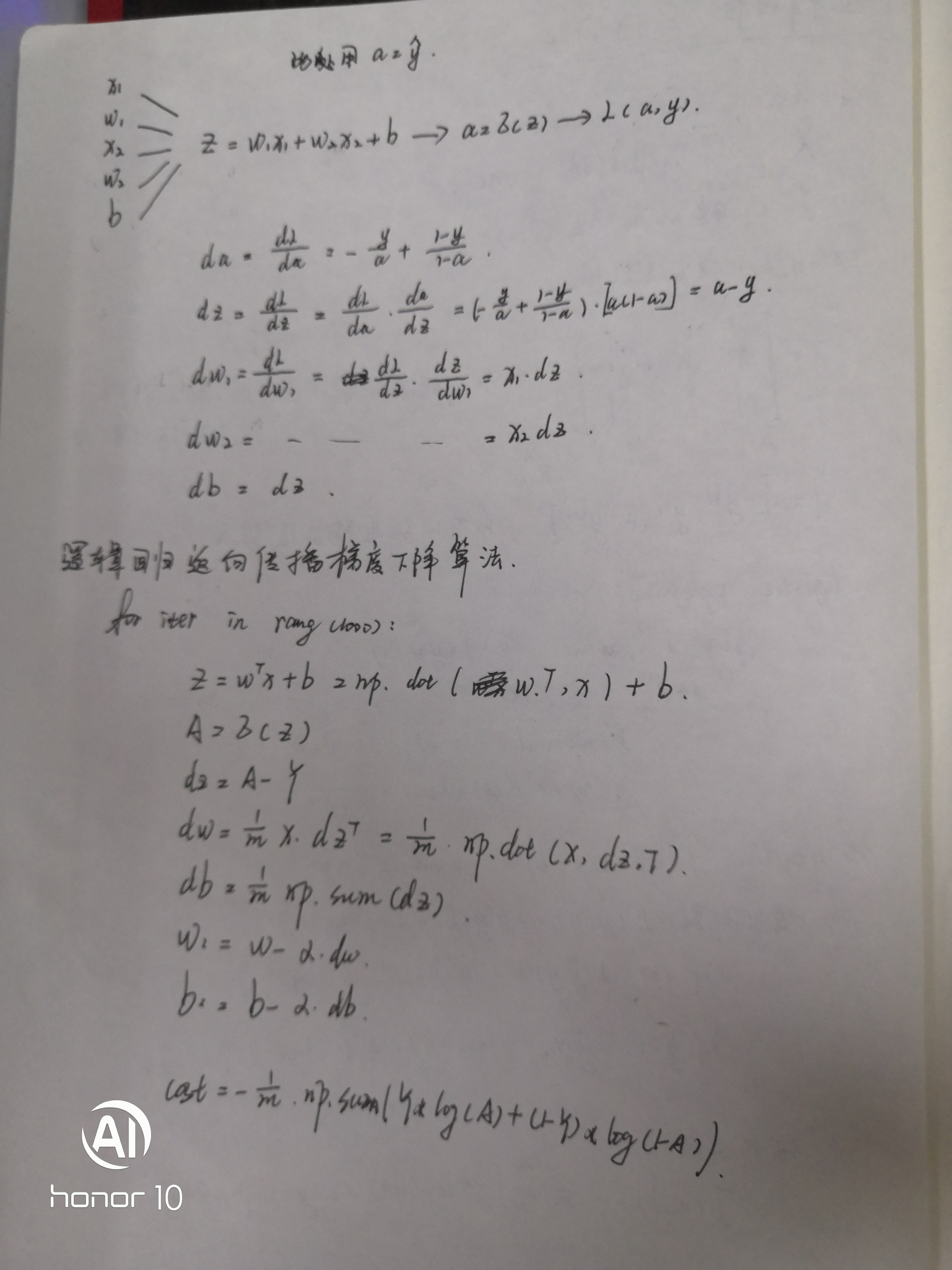## 4. 损失函数和凸函数之间的关系

损失函数，即判断这个网络性能的函数，表示为L(a,y)

## 5. 全局最优和局部最优

全局最优，理解为整个的最优化，局部最优，找到一个某个范围的最优化参数

参考问题3

## 8. L2-Norm、L1-Norm、L0-Norm

L0范数是指向量中非0的元素的个数
L1范数是指向量中各个元素绝对值之和，又称曼哈顿距离
L2范数: ||W||2。就是欧几里德距离

## 9. 正则化

L2正则化，预防过拟合，减少方差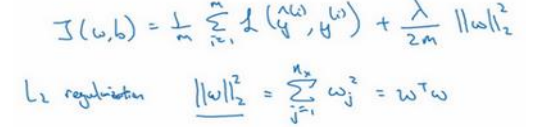李宏毅机器学习 相关内容

阅读数 3254

博文 来自： cp_oldy

阅读数 19

博文 来自： weixin_43369974
博文 来自： jackjia1994
博文 来自： lonnee
博文 来自： qq_36459893
没有更多推荐了，返回首页